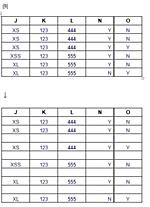人力検索はてな
ﾓﾊﾞｲﾙ版を表示しています｡PC版はこちら空白行挿入のｴｸｾﾙﾏｸﾛの作成をお願いします｡

A列?X列までﾃﾞｰﾀが入っています｡
1行目は見出し行です｡●拡大する

●質問者: tororosoba
●ｶﾃｺﾞﾘ:ｺﾝﾋﾟｭｰﾀ
○ 状態 :終了
└ 回答数 : 1/1件

▽最新の回答へ

 ▽1 ● a-kuma3 ●800ﾎﾟｲﾝﾄﾍﾞｽﾄｱﾝｻｰ

こんな感じでどうでしょう｡

```Sub insert_row()
Dim prev
col_s = 10  ' J列
col_e = 14  ' N列
ReDim prev(col_s To col_e)

last_row = Cells(Rows.Count, 10).End(xlUp).Row
For c = col_s To col_e
prev(c) = Cells(last_row, c).Value
Next

For r = last_row - 1 To 1 Step -1
For c = col_s To col_e
If Cells(r, c).Value <> prev(c) Then
Rows(r + 1).Insert
Exit For
End If
Next
For c = col_s To col_e
prev(c) = Cells(r, c).Value
Next
Next
End Sub
```

J?N列には､空白のｾﾙがない前提です｡

M列が比較の対象外ということに気が付きませんでした m(_ _)m
M列を比較の対象外にしたｺｰﾄﾞは以下のようになります｡

```Sub insert_row()
Dim prev
col_s = 10  ' J列
col_e = 15  ' O列
ReDim prev(col_s To col_e)

last_row = Cells(Rows.Count, 10).End(xlUp).Row
For c = col_s To col_e
prev(c) = Cells(last_row, c).Value
Next

For r = last_row - 1 To 1 Step -1
For c = col_s To col_e
If c <> 13 And Cells(r, c).Value <> prev(c) Then
Rows(r + 1).Insert
Exit For
End If
Next
For c = col_s To col_e
prev(c) = Cells(r, c).Value
Next
Next
End Sub
```

tororosobaさんのｺﾒﾝﾄ
ありがとうございます｡ 使わせていただいたのですが､二行目以降から一行ごとに空白行が挿入されてしまうようです｡JKLMNのﾃﾞｰﾀ重複が途切れた各箇所で空白行を挿入するのは無理でしょうか｡

a-kuma3さんのｺﾒﾝﾄ

tororosobaさんのｺﾒﾝﾄ
M列を削除してﾏｸﾛを実行してみたら添付のような結果になったのですが､非対象列のM列を残したままこのﾏｸﾛを使うことはできないでしょうか？

a-kuma3さんのｺﾒﾝﾄ
あ､M列は対象外なんですね｡ 直します｡

a-kuma3さんのｺﾒﾝﾄ

tororosobaさんのｺﾒﾝﾄ
お手を煩わせて申し訳ございませんでした｡ 希望通りに動きました！ 本当に助かりました､ありがとうございます！
 関連質問

●質問をもっと探す●

0.人力検索はてなﾄｯﾌﾟ
8.このﾍﾟｰｼﾞを友達に紹介
9.このﾍﾟｰｼﾞの先頭へ

お問い合わせ
ﾍﾙﾌﾟ/お知らせ
ﾛｸﾞｲﾝ

はてなﾄｯﾌﾟ